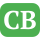CppBuzz.com

# rectangle.c -CppBuzz-Forum

```Hi i have question did u know how to to e Write a function rectangle that calculates and returns the area of ​​a rectangle whose both sides (integers) are passed as parameters of the function.  and then Test your function by calling it from within the function  main and then issuing the Run command.

Here some of my code
----------------------

int main (void){
/* code to verify if the function works as expected */
int a=10, b=3;
int res = rectangle(a,b);
printf("rectangle(%d, %d) = %d\n", a, b, res);
return EXIT_SUCCESS;
}

Hope u guy help me ```

Posted by Cstudents 2021-02-07 08:50:17

```int rectangle(int a, int b)
{
return a*b;
}

int main (void){
/* code to verify if the function works as expected */
int a=10, b=3;
int res = rectangle(a,b);
printf("rectangle(%d, %d) = %d
", a, b, res);
return EXIT_SUCCESS;
}```Admin posted 2021-02-07 09:55:18

```Thank Admin for your help now i understand
Thank again.```Cstudents posted 2021-02-07 10:32:30

Please login to comment